# Real number calculator

The calculator makes basic and advanced operations with real numbers, natural numbers, integers, rational and irrational numbers. It also shows detailed step-by-step information about calculation procedure. Solve problems with two, three, or more real numbers in one expression. Add, subtract, and multiply real numbers step-by-step. This calculator performs addition, subtraction, multiplication, or division for calculations on positive or negative real numbers. This online real number calculator will help you understand how to add, subtract, multiply, or divide real numbers.

Real numbers are numbers that can be found on the number line. This includes the natural numbers( 1,2,3 ...), integers (-3) rational (fractions) and irrational numbers (like √2 or π). Positive or negative, large or small, whole numbers or decimal numbers are all real numbers. Imaginary numbers and complex numbers cannot be draw in number line, but in complex plane.

#### Examples:

addition of real numbers: -3/2 + pi/2
subtraction of real numbers: 32.14 - 67.32
parenthesis: sin(pi/2) * (1.1+2.2+3.3)
Complex example: Priority of operators and brackets: 3⋅[8−(−1) + (−3)÷(−3)]^2

## Real numbers in word problems:

• Evaluate 18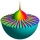Evaluate the expression (-4-7i)-(-6-9i) and write the result in the form a+bi (Real + i* Imaginary).
• Complex plane mapping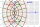Show that the mapping w = z +c/z, where z = x+iy, w = u+iv and c is a real number, maps the circle |z| = 1 in the z-plane into an ellipse in the (u, v) plane.
• The diameter 2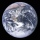The diameter of the Earth is 12,756 kilometers. If Mercury's diameter is about 7,876.6 kilometers shorter than that of the Earth, what is the diameter of Mercury?
• Is complexAre these numbers 2i, 4i, 2i + 1, 8i, 2i + 3, 4 + 7i, 8i, 8i + 4, 5i, 6i, 3i complex?
• Is equal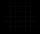Is equal following terms? -9 21 = (-9) 21
• Decimal number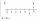I am a decimal number between 0.3 and 0.5 . the digit in my hundredths place is 5 more than the digit in tenth place, off the number in tenth place is 4. what is the number?
• Imaginary numbersFind two imaginary numbers whose sum is a real number. How are the two imaginary numbers related? What is its sum?
• Fruit punch 2Alberto makes fruit punch with 24.2 ounces of apple juice and 18.4 ounces of pineapple juice. He splits the fruit punch equally into 6 glasses. How much fruit punch is in each glass?
• Write 3Write a real-world problem involving the multiplication of a fraction and a whole number with a product that is between 8 and 10, then solve the problem.
• Two midpoints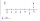Point A is on a number line halfway between -17 and 5 . Point B is halfway between Point A and 0. What integer does Point B represent?
• The sum 15The sum of the real numbers x and y is 24. Their difference is 12. What is the value of xy?
• On the number lineWhat number is exactly in the middle between 9.8 and -2.4 on the number line?
• Which 8Which among the following real numbers below is the least? A. 5/8 B. 0.75 C. 7/10 D. 35/99
• Equation with abs value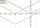How many solutions has the equation (|x| +x) |x-3| = |x+1| in the real numbers?
• Subtract 4Subtract the following decimals. a. 0.98 - 0.053 b. 0.67 - 0.4 c. 0.3 - 0.002 d. 3.2 - .789 e. 6.53 - 4.298 f. 6 - 4.32 g. 7 - 3.574 h. 4.83 - 1.8 i. 3.7 - 1.8 j. 16.17 - 11.632
• Logarithmic equation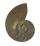Solve equation: log13(7x + 12) = 0
• Sum and productThe sum of the real numbers x and y is 12. Their difference is 8. What is the value of xy?